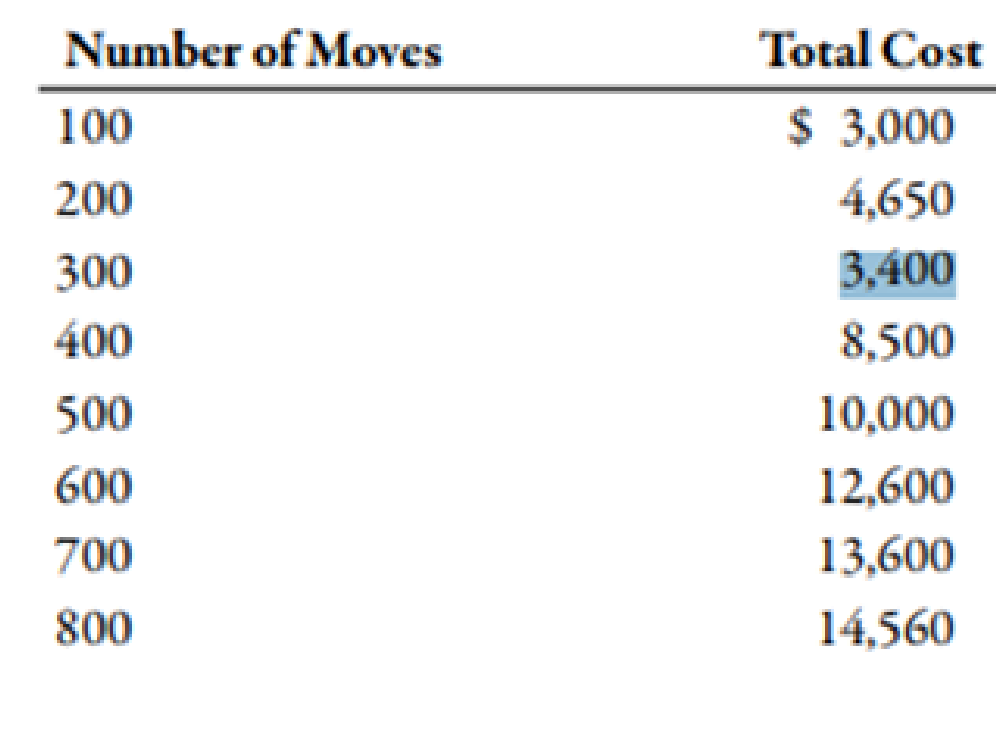# ( Appendix 3A) Separating Fixed and Variable Costs, Service Setting Louise McDermott, controller for the Galvin plant of Veromar Inc., wanted to determine the cost behavior of moving materials throughout the plant. She accumulated the following data on the number of moves (from 100 to 800 in increments of 100) and the total cost of moving materials at those levels of moves: Required: 1. Prepare a scattergraph based on these data. Use cost for the vertical axis and number of moves for the horizontal axis. Based on an examination of the scattergraph, does there appear to be a linear relationship between the total cost of moving materials and the number of moves? 2. Compute the cost formula for moving materials by using the high-low method. Calculate the predicted cost for a month with 550 moves by using the high-low formula. ( Note: Round the answer for the variable rate to three decimal places and the answer for total fixed cost and total cost to the nearest dollar.) 3. CONCEPTUAL CONNECTION Compute the cost formula for moving materials using the method of least squares. ( Note: For the method of least squares, round the variable rate to two decimal places and total fixed cost and total cost to the nearest dollar.) Using the regression cost formula, what is the predicted cost for a month with 550 moves? What does the coefficient of determination tell you about the cost formula computed by regression? 4. CONCEPTUAL CONNECTION Evaluate the cost formula using the least squares coefficients. Could it be improved? Try dropping the third data point (300, $3,400), and rerun the regression.BuyFind ### Managerial Accounting: The Corners... 7th Edition Maryanne M. Mowen + 2 others Publisher: Cengage Learning ISBN: 9781337115773BuyFind ### Managerial Accounting: The Corners... 7th Edition Maryanne M. Mowen + 2 others Publisher: Cengage Learning ISBN: 9781337115773 #### Solutions Chapter Section Chapter 3, Problem 69P Textbook Problem 323 views ## (Appendix 3A) Separating Fixed and Variable Costs, Service SettingLouise McDermott, controller for the Galvin plant of Veromar Inc., wanted to determine the cost behavior of moving materials throughout the plant. She accumulated the following data on the number of moves (from 100 to 800 in increments of 100) and the total cost of moving materials at those levels of moves:Required: 1. Prepare a scattergraph based on these data. Use cost for the vertical axis and number of moves for the horizontal axis. Based on an examination of the scattergraph, does there appear to be a linear relationship between the total cost of moving materials and the number of moves? 2. Compute the cost formula for moving materials by using the high-low method. Calculate the predicted cost for a month with 550 moves by using the high-low formula. (Note: Round the answer for the variable rate to three decimal places and the answer for total fixed cost and total cost to the nearest dollar.) 3. CONCEPTUAL CONNECTION Compute the cost formula for moving materials using the method of least squares. (Note: For the method of least squares, round the variable rate to two decimal places and total fixed cost and total cost to the nearest dollar.) Using the regression cost formula, what is the predicted cost for a month with 550 moves? What does the coefficient of determination tell you about the cost formula computed by regression? 4. CONCEPTUAL CONNECTION Evaluate the cost formula using the least squares coefficients. Could it be improved? Try dropping the third data point (300,$3,400), and rerun the regression.

Expert Solution

1.

To determine

Construct the scatter graph by plotting the number of moves and total cost. Also, identify the relationship between the number of moves and total cost.

### Explanation of Solution

Scatter Graph Method:

In a scatter graph method, data points are plotted on the graph to visualize the relationship between total cost and output measure.

Expert Solution

2.

To determine

Construct the cost formula for moving materials with the help of high-low cost method.

Expert Solution

3.

To determine

Construct the cost formula for moving materials with the help of lease square method and determine the values of fixed cost and variable rate with the help of excel.

Expert Solution

4.

To determine

Construct the cost formula with the help of least square coefficients.

### Want to see the full answer?

Check out a sample textbook solution.See solution

### Want to see this answer and more?

Bartleby provides explanations to thousands of textbook problems written by our experts, many with advanced degrees!

See solution